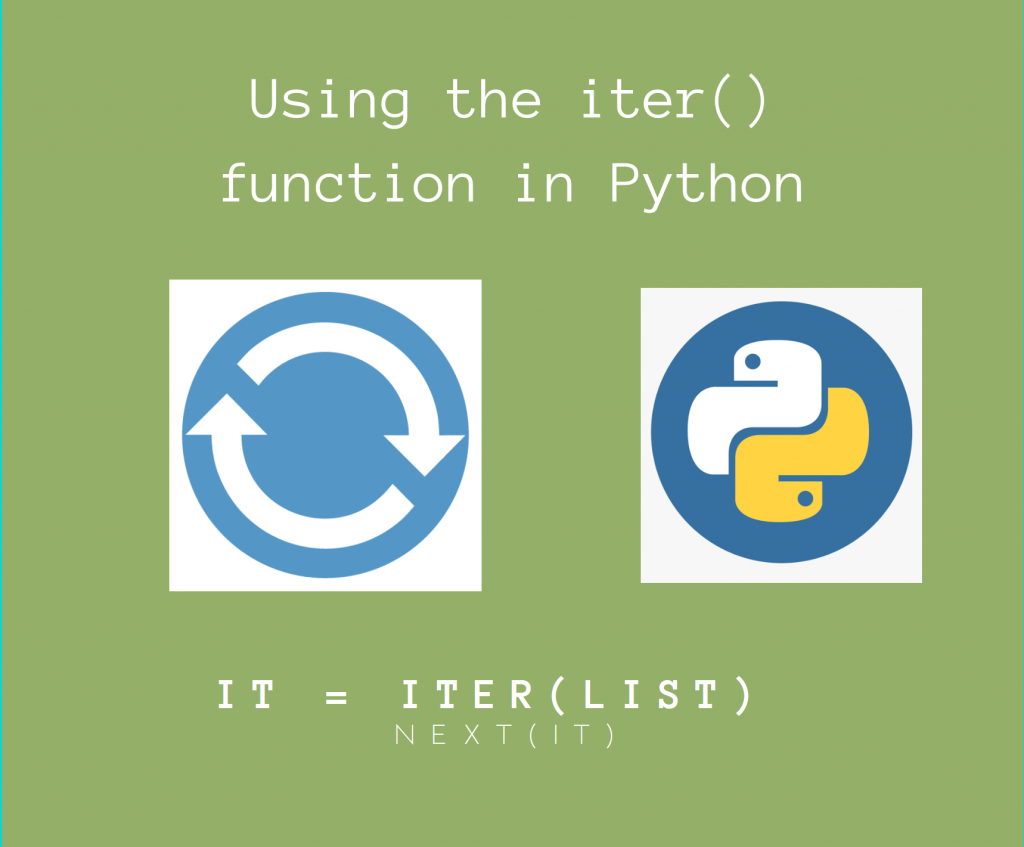# How to Use the Python iter() Method?In this article, we’ll take a look at using the Python iter() function.

Often, we may want to use iterators, which is an object which itself loads Python objects. But, as opposed to an array or a list, an iterator simply loads objects only as and when required.

## Basic Syntax of Python iter()

We can use the `iter()` function to generate an iterator to an iterable object, such as a dictionary, list, set, etc.

The basic syntax of using the Python `iter()` function is as follows:

```iterator = iter(iterable)
```

This will generate an `iterator` from the `iterable` object.

We can simply load objects one by one using `next(iterator)`, until we get the `StopIteration` Exception.

Also, note that we CANNOT iterate through the iterable again using the same iterator. We must generate another iterator using Python `iter()` before iterating!

## Using Python iter() – A simple Example

Here is a simple example using `iter()`. We’ll take a list of 10 elements and load them one-by-one.

```a = [i for i in range(10)]

iterator = iter(a)

while True:
try:
out = next(iterator) # Load the next element
except StopIteration:
break
```

Output

```Iterator loaded 0
```

As you can see, indeed, it loads elements from the list one by one, until we catch the `StopIteration` Exception!

## Using Python iter() for custom objects

As I mentioned earlier, we can use Python iter() on any object, provided that it is iterable.

This applies to custom objects as well, provided it satisfies a few conditions.

But what are the conditions for any object to be an iterable in Python?

• The class of that object must have the `__iter__()` method.
• The class of the object must have the `__next__()` method. Also, it is suggested that you also raise a `StopIteration` Exception if the terminating condition is reached.

Now, the Python `iter()` method will construct the iterator and call the `__iter__()` method. Similarly, the `next(iterator)` will call the `__next__()` method behind the hood.

NOTE: If the class does NOT have these methods, it must atleast have the `__getitem()__` method, with integer arguments from 0. Otherwise, we will get a `TypeError` Exception.

Let’s now write a class for a custom object, which generates integers until a limit.

```class MyClass():
def __init__(self, max_val):
# Set the maximum limit (condition)
# max_val must be a natural number
assert isinstance(max_val, int) and max_val >= 0
self.max_val = max_val
def __iter__(self):
# Called when the iterator is generated
# Initialise the value to 0
self.value = 0
return self
def __next__(self):
# Called when we do next(iterator)
if self.value >= self.max_val:
# Terminating condition
raise StopIteration
self.value += 1
# Return the previously current value
return self.value - 1

# Set the limit to 10
my_obj = MyClass(10)

# An iterator to the object
my_iterator = iter(my_obj)

while True:
try:
val = next(my_obj)
except StopIteration:
break
```

Output

```Iterator Loaded 0
```

As you can see, we are indeed able to use the `iter()` function on our custom object. The `__iter__()` method creates the iterator object, which we then update using `__next__()`.

The terminating condition is when the current value is greater than the maximum value, which is when we raise a `StopIteration` exception.

## Generate values until a sentinel value with iter()

We can pass one more argument to Python `iter()`. This second argument is called the `sentinel` element.

If we pass this sentinel element, the iterator will keep generating values until the generated value equals this sentinel value, after which `StopIteration` will be raised.

After this, the iterator generation will automatically stop!

This is very useful if you have sequential data coming from functions. Functions are also necessary, since the first argument MUST be callable if we use the sentinel argument.

```iterator = iter(callable_object, sentinel)
```

Here, `iterator` is an iterator which will keep calling `callable_object` until the returned value equals `sentinel`.

Here, `callable_object` can be a function, method, or even a Lambda!

Let’s take a simple example using a Lambda as a callable.

We’ll take a string as input, and pass it to a lambda function, and keep generating values until a newline sentinel element (‘\n’).

```a = "This is a long string consisting of two lines.\nThis is the second line.\nThis is the third line."

start = 0
size = 1

def func(a):
return a[start: start+size]

iterator = iter(lambda: func(a), '\n')

# Will generate values until '\n'
for out in iterator:
start += size

print("Encountered Newline!")
```

Output

```Iterator loaded T
As you can observe, the iterator generate values until it encountered a newline! You could also have done the same program using a `while` loop and catching the `StopIteration` exception.
This is actually very useful if you want to deal with blocks of outputs returned by functions, so definitely be aware of the sentinel parameter to `iter()`!
In this article, we looked at how we could use the `iter()` function in Python to generate iterables for various objects.